## Rational Point

A-rational point is a pointon an Algebraic Curve, whereandare in a Field.

The rational point may also be a Point at Infinity. For example, take the Elliptic Curveand homogenize it by introducing a third variableso that each term has degree 3 as follows: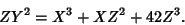Now, find the points at infinity by setting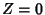, obtainingSolving gives,equal to any value, and (by definition). Despite freedom in the choice of, there is only a single Point at Infinity because the two triples (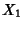,,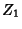), (,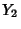,) are considered to be equivalent (or identified) only if one is a scalar multiple of the other. Here, (0, 0, 0) is not considered to be a valid point. The triples (,, 1) correspond to the ordinary points (,), and the triples (,, 0) correspond to the Points at Infinity, usually called the Line at Infinity.

The rational points on Elliptic Curves over the Galois Field GF(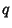) are 5, 7, 9, 10, 13, 14, 16, ... (Sloane's A005523).

Sloane, N. J. A. Sequence A005523/M3757 in An On-Line Version of the Encyclopedia of Integer Sequences.'' http://www.research.att.com/~njas/sequences/eisonline.html and Sloane, N. J. A. and Plouffe, S. The Encyclopedia of Integer Sequences. San Diego: Academic Press, 1995.